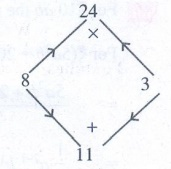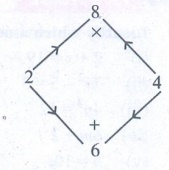Home | | Maths 8th Std | Exercise 3.5

# Exercise 3.5

8th Maths : Chapter 3 : Algebra : Miscellaneous Practice problems, Challenge Problems, Text Book Back Exercises Questions with Answers, Solution

Exercise 3.5

Miscellaneous Practice Problems

1. Subtract: 2(xy)2 ( y 3 + 7x2 y + 5) from 5y 2 (x2 y 3 2x4 y + 10x2 )

Solution:

5y2 (x2 y3 − 2x4y + 10x2) − [(−2) (xy)2 (y3 + 7x2y + 5)]

= [5y2 (x2 y3) − 5y2 (2x4 y) + 5y2 (10x2)] − [(−2) x2y2 (y3 + 7x2 y + 5)]

= (5y5x2 − 10 x4y3 + 50 x2y2) − [(−2x2y2) (y3) + (−2x2y2) (7x2y) + (−2x2y2) (5)]

= 5x2y5 − 10x4y3 + 50x2y2 − [−2x2 y5 − 14x4y3 − 10x2y2]

= 5x2y5 − 10x4y3 + 50x2y2 + 2x2 y5 + 14x4y3 + 10x2y2

= (5 + 2) x2y5 + (−10 + 14) x4y3 + (+50 + 10)x2y2

= 7x2y5 + 4x4y3 + 60x2y2

2. Multiply ( 4x2 + 9) and ( 3x 2)

Solution:

(4x2 + 9) (3x − 2) = 4x2 (3x − 2) + 9 (3x − 2)

= (4x2) (3x) − (4x2) (2) + 9 (3x) − 9(2) = (4 × 3 × x × x2) − (4 × 2 × x2) + (9 × 3 × x) − 18

= 12x3 − 8x2 + 27x − 18 (4x3 + 9) (3x− 2) = 12x3 − 8x2 + 27x − 18

3. Find the simple interest on Rs. 5a 2b2 for 4ab years at 7b% per annum.

Solution:Simple interest = [ Principal × years ×  rate of interest ] / 100

= [ 5a2b2 × 4ab × 7b ] / 100 = [ (5 × 4 × 7) (a2 × b2 × a × b × b) ] / 100

= (140/100) (a3 b4) = (14/10)  a3 b4

Simple Interest = (7/5) a3 b4

4. The cost of a note book is Rs. 10ab. If Babu has Rs. ( 5a 2b + 20ab 2 + 40ab) . Then how many note books can he buy?

Solution:

For ₹10 ab the number of note books can buy = 1.

For ₹ (5a2b + 20ab2 + 40 ab) the number of note books can buy = Total amount / cost of 1 note book= [ 5a2b + 20ab2 + 40ab ] / 10 ab = [ 5a2b / 10ab ] + [ 20ab2 / 10ab ] + [ 40ab / 10ab ]

= 1/2 [  a2−1 b1−1 ] + [ 2 a1−1 b2−1 ] + [ 4 a1−1 b1−1 ] = (1/2)a1 b0 + 2 a0 b1 + 4 a0 b0

Number of note books he can buy = (½)a + 2b + 4

5. Factorise : ( 7y2 19y 6)

Solution:

7y2 − 19y – 6 is of the form ax2 + bx + c where a = 7; b = −19; c = − 6

Product = −42 : Sum = −19

1 × −42 = −42 : 1 + (−42) = −41

2 × −21 = −42 : 2 + (−21) = −19

The product a × c = 7x − 6 = − 42

sum b = −19The middle term − 19y can be written as − 21y + 2y

7y2 − 19y − 6 = 7y2 − 21y + 2y − 6

= 7y (y − 3) + 2 (y − 3) = (y − 3) (7y + 2)

7y2 − 19y − 6 = (y − 3) (7y + 2)

Challenging problems

6. A contractor uses the expression 4x2 + 11x + 6 to determine the amount of wire to order when wiring a house. If the expression comes from multiplying the number of rooms times the number of outlets and he knows the number of rooms to be ( x + 2), find the number of outlets in terms of ‘x’. [Hint : factorise 4x 2 + 11x + 6 ]

Solution:

Given Number of rooms = x + 2

Number of rooms × Number of outlets = amount of wire.

(x + 2) × Number of outlets = 4x2 + 11x + 6

Number of outlets = [ 4x2 + 11x + 6 ] / [ x + 2]         ...(1)

Now factorising 4x2 + 11x + 6 which is of the form ax2 + bx + c with a = 4 b = 11 c = 6.

The product a × c = 4 × 6 = 24

sum b = 11

Product = 24 : Sum = 11

1 × 24 = 24    : 1 + 24 = 25

2 × 12 = 24    :  2 + 12 = 14

3 × 8 = 24      :  3 + 8 = 11The middle term 11x can be written as 8x + 3x

4x2 + 11x + 6 = 4x2 + 8x + 3x + 6

= 4x (x + 2) + 3 (x + 2)

4x2 + 11x + 6 = (x + 2) (4x + 3)

Now from (1) the number of outlets[ 4x2 + 11x + 6 ] / [ x + 2] = [ (x + 2) (4x + 3)] / (x + 2)    = 4x + 3

∴  Number of outlets = 4x + 3

7. A mason uses the expression x2 + 6x + 8 to represent the area of the floor of a room. If the decides that the length of the room will be represented by ( x + 4) , what will the width of the room be in terms of x ?

Solution:

Given length of the room = x + 4

Area of the room = x2 + 6x + 8 = x2 + 6x + 8

Length × breadth = x2 + 6x + 8

Breadth = [ x2 + 6x + 8 ] / [ x + 4 ]      ……(1)

Factorizing x2 + 6x + 8, it is in the form of ax2 + bx + c

Where a = 1 ; b = 6 ; c = 8;

The product a × c = 1 × 8 = 8

Sum = 6

Product = 8 : Sum = 6

1 × 8 = 8   :  1 + 8 = 9

2 × 4 = 8   :  2 + 4 = 6The middle term 6x can be written as 2x + 4x

x2 + 6x + 8 = x2 + 2x + 4x + 8

= x (x + 2) + 4 (x + 2)

x2 + 6x + 8 = (x + 2) (x + 4)

Now from (1)

breadth = [ x2 + 6x + 8 ] / (x + 4) = [ (x + 2) (x + 4) ] / (x + 4) = (x + 2)

∴  Width of the room = x + 2

8. Find the missing term: y2 + (…) x + 56 = (y+7)(y+..)

Solution:

We have (x + a) (x + b) = x2 + (a + b)x + ab

56 = 7 × 8

∴  y2 + (7 + 8)x + 56 = (y + 7) (y + 8)

9. Factorise : 16 p4 1

Solution:

16p4 1 = 24 p4 − 1 = (22)2 (p2)2 − 12

= (22p2)2 − 12

Comparing with a2b2 = (a + b) (a − b) where a = 22 p2 and b = 1

(22p2)2 − 12 = (22p2 + 1) (22p2 − 1)

= (4p2 + 1) (4p2 − 1)

16p4 − l = (4p2 + 1) (4p2 − 1) = (4p2 + 1) (22p2 − 12)

= (4p2 + 1) [(2p)2 − 12] = (4p2 + 1) (2p + l) (2p − 1)

[ using a2b2 = (a + b) (a − b)]

16p4 − 1 = (4p2 + 1) (2p + l) (2p − 1)

10. Factorise : 3x3 – 45x2y + 225xy2 – 375y3

= 3x3 − 45x2y + 225xy2 − 375y3

= 3(x3 − 15x2y + 75xy2 − 125y3)

= 3(x3 − 3x2(5y) + 3x(5y)2 − (5y)3)

= 3(x − 5y)3

Exercise 3.5

Miscellaneous Practice Problems

1. 7x2y5 + 4 x4y3 + 60x2 y2

2.12 x3 − 8x2 + 27x −18

3. S.I = 7/5 a3b4

4. 1/2 a + 2b + 4

5. ( y − 3)(7y + 2)

Challenging Problems

6. 4x + 3

7. x + 2

8. ( y + 7)( y + 8)

9. (4 p2 + 1)( 2p +1)(2p −1)

10. 3( x − 5y)3

Tags : Questions with Answers, Solution | Algebra | Chapter 3 | 8th Maths , 8th Maths : Chapter 3 : Algebra
Study Material, Lecturing Notes, Assignment, Reference, Wiki description explanation, brief detail
8th Maths : Chapter 3 : Algebra : Exercise 3.5 | Questions with Answers, Solution | Algebra | Chapter 3 | 8th Maths